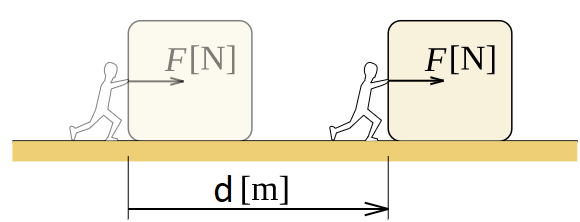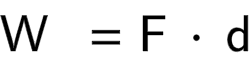# Work Calculator

Created by Álvaro Díez
Reviewed by Dominik Czernia, PhD candidate and Jack Bowater
Last updated: Apr 14, 2022

Welcome to the work calculator, where you can learn the work formula, and how to calculate work. For physicists, work is a very different concept than in normal life, there's even a work equation!

Come in to read about the work definition, and what are SI units for work. It might seem complicated, but I promise it won't be much "work".

If you came here to calculate work done by gases, then our combined gas law calculator is the tool you are looking for.

## Work definition in physics and work formula

In our everyday life, work is any kind of effort, job, or action that we perform. Work in physics, however, has a very strict and clear definition. As it happens normally, the work definition in physics is almost the same as the work equation.In physics, to have work we need an object to move as a result of a force applied to it. The work equation (or the work definition if you will) is:

W = F * d

where,

• W is the work done;
• F is the force applied to the object; and
• d is the distance about which you moved the object.

The force can be decomposed like this:

F = m * a

where,

• m is the mass of the object; and
• a the acceleration it experiences.

Remember that when the object is being lifted at a constant speed (a typical school problem), the acceleration on the object is g (the gravitational force).

Work and power are two related concepts, you can calculate the power (P) from the work done if you know the time (t) that it took to do such work. Simply use the equation:

P = W / t

## Work from velocity change

If we want to go down the rabbit hole, we can use any force formula we want. Then substitute the value into the work equation, and get the result. Other times, that might be an unnecessary step.

Consider an object that changes its speed from an initial velocity (v0) to a final velocity (v1) over a time (t). We could calculate the force using the acceleration:

F = m * a = m * (v1 - v0) / t

then we obtain the distance traveled that can be estimated as:

d = t * (v0 + v1) / 2

and then combine everything into the work formula:

W = F * d = [m * (v1 - v0) / t] * [t * (v0 + v1) / 2]

W = (m/2) * (v12 - v02)

## SI units of work in physics

There are many instances in which the units used in real-life don't match the units used in physics, but work is not one of those... kind of. If you look at the work formula, you can see that the units are [Newtons]*[meters] for the SI system. The SI units of work are, therefore, Joules.

Joules (and calories) are typically called units of energy, but energy and work use the same units, as they are very similar concepts in physics.

## Work equation - how to calculate work?

If all you came here for is learn how to calculate work, this is your section. You are doubly lucky since the calculation is fairly simple, as you might have guessed from the work formula.

All you need to do is take the force, F, and multiply it by the distance the object moved. The result will be the work performed on the object.

If you want to get fancy/advanced, you can include the force definition into the work equation and obtain:

W = m * a * d

which is still a simple multiplication that can be done quickly. Too much work for you? Don't fret! Our calculator will tell you exactly how much work has been done in your scenario, so you can save that precious brainpower.

## How to use the work calculator?

The work calculator is very simple to use. In its basic form, you simply need to input the force and the distance from your scenario, and it will automatically calculate the result for you.

If you are an adventurous person, you can use the advanced mode to get to more... advanced calculations and alternative modes.

When you enter this mode, you will see 3 different sections that will allow you to calculate:

• Calculate work from mass, initial velocity, and final velocity;
• Calculate work from Force and distance;
• Calculate Force from mass and acceleration;
• Calculate Acceleration from initial velocity, final velocity and time;
• Calculate Power from work and time; and...
• Many more!

This work calculator is smart. All you need to do is input the values you know and it will do calculate the work and all other possible values for you!

## FAQ

### How do I calculate work done by force?

To calculate work done by a force, follow the given instructions:

1. Find out the force, F, acting on an object.

2. Determine the displacement, d, caused when the force acts on the object.

3. Multiply the applied force, F, by the displacement, d, to get the work done.

### How do I calculate distance with force and work?

The formula for calculating work is Work = Force x Distance. Hence, to calculate the distance from force and work, proceed as follows:

1. Determine the work done, W, when the force, F, is applied.

2. Divide the work done, W, by the applied force, F.

3. Congrats, you have calculated the distance from force and work!

### How do I calculate the work done to an object at an angle?

To calculate the work done to an object at an angle, follow the given instructions:

1. Find out the magnitude of the applied force, F, and the distance traveled, d, by the object.

2. Calculate the cosine of the angle θ, which is between the force and distance vectors.

3. Multiply the force, F, distance, d, and the cosine of the angle,  cos θ, together to get the work done to an object at an angle.

### Under what conditions is work done zero?

The work done is zero when one or more of the following conditions are satisfied:

1. The force applied is zero;
2. The displacement is zero; and
3. The angle between the force and displacement vectors is zero.
Álvaro DíezCalculate from
force and displacement
Work calculation
Force (F)
N
Displacement (d)
yd
Work (W)
J
People also viewed…

### Circle skirt

Circle skirt calculator makes sewing circle skirts a breeze.

### Cyclotron Frequency

Use the Cyclotrone Frequency Calculator to explore the world of the first particle's accelerators.

### Manometer

This manometer calculator will determine pressures at specific points in any common manometer. Manometers are simple to use and knowing how to use them is a fantastic way to learn more about fluid statics.

### Significant figures

The significant figures calculator performs operations on sig figs and shows you a step-by-step solution!